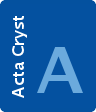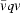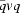## book reviewsFOUNDATIONSADVANCES
ISSN: 2053-2733

On quaternions and octonions: their geometry, arithmetic and symmetry . By John H. Conway and Derek A. Smith. Natick, MA: A. K. Peters Ltd, 2003. Price USD 29.00. ISBN 1-56881-134-9

aLaboratory for Neutron Scattering, ETH Zürich and Paul Scherrer Institut, CH-5232 Villigen PSI, Switzerland
*Correspondence e-mail: hans.grimmer@psi.ch

(Received 20 February 2002; accepted 16 May 2003)

This book consists of three chapters of increasing length, dealing with complex numbers, quaternions and octonions, respectively. I shall concentrate my review on the first two chapters, where the connection with concepts familiar to crystallographers is made.

In the introductory chapter on complex numbers, the authors show that the complex units correspond to rotations in two-dimensional space and they determine the finite subgroups of the special and general orthog­onal group in two dimensions. Then they discuss definitions of complex integers with unique factorization into products of primes, the simplest of which, Gaussian and Eisenstein integers, correspond to the square and hexagonal lattice, respectively. Finally, they reproduce Polya's famous illustration of the 17 types of two-dimensional space groups. Instead of the usual symbols for these groups, `orbifold symbols' are given, of which only a summary definition appears in the book.

Whereas for complex numbers the terms norm, unit and complex conjugate are defined, they are taken for granted in the case of quaternions. It is shown that there exists a 2-to-1 homomorphism from the group of unit quaternions to the group SO3 of rotations in 3-dimensional space. The finite subgroups of SO3 are derived using spherical trigonometry, similar to that done in the book Geometric Symmetry by Lockwood & Macmillan (Cambridge University Press, 1978). The transition to the finite subgroups of the group of unit quaternions is mentioned only briefly. More space is devoted to the derivation and listing of the finite subgroups of the general orthogonal group GO3. These are the well known (generally non-crystallographic) point groups listed also in Volume A of International Tables for Crystallography. Various kinds of symbols are used to denote these point groups, one resembles the Schoenflies notation whereas the orbifold symbols resemble the Hermann–Mauguin notation.

It is then shown that a 2-to-1 homomorphism exists between ordered pairs of unit quaternions and SO4. Names characterizing the finite subgroups of SO4 are introduced; in the case of polyhedral groups, also modified Coxeter symbols are given. The authors claim that they have for the first time given unique names to all finite subgroups of SO4. They explain the various types of chirality shown by subgroups of SO4. Finally, Hurwitz and Lipschitz integral quaternions are defined and their factorization into products of primes is discussed.

The book has been written by two mathematicians. A great advantage is that it is written in a style that makes it easily accessible also to other scientists. The main messages are clearly stated, not buried under technical details. The book is carefully written; I noted only few misprints: In Figure 2.1, z03 and zz02 are shown instead of z02 and zz0; the orbifold symbols 3*2 and *332 are exchanged in Table 3.1; the alternative symbols for 2*12 and 2*30 have wrong subscripts in Table 3.2:should be replaced byin the title of the Appendix on page 40.

The authors do not state for what readership they have written the book. Although it cannot serve as a basic text for crystallographers, I enjoyed reading it and added Hermann–Mauguin symbols to many tables and figures. Looking at the title of the book, I had hoped that it might also discuss the use of quaternions to derive the coincidence misorientations of cubic lattices, which is not the case. Instead it gives the17 types of two-dimensional space groups and the finite subgroups of GO3, which seem to me less closely related to quaternions.

Follow Acta Cryst. A